Select PageThe Grade 5 Math Workbook has lots of exercises. The workbook consists of 143 pages. The book is written according to the CAPS curriculum.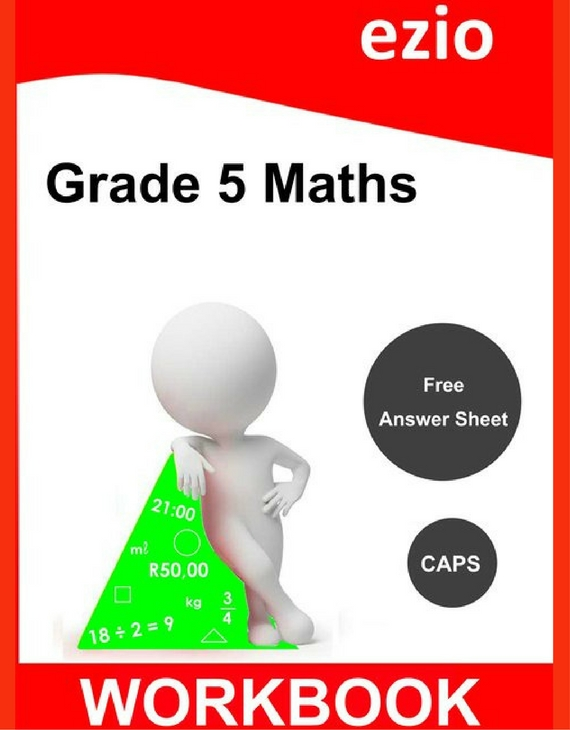Ezio Grade 5 Workbook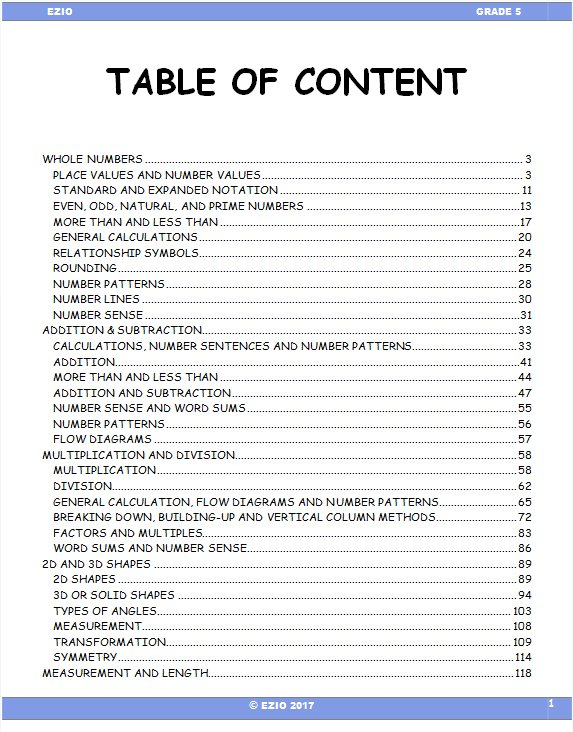Whole Numbers
Multiplication & Division
2D and 3D Shapes
Measurement – Length
Measurement – Mass
Measurement – Capacity
Time
Circumference, Area, Volume
Fractions
Money
Ratio and Proportion
Data Handling
Coordinate grids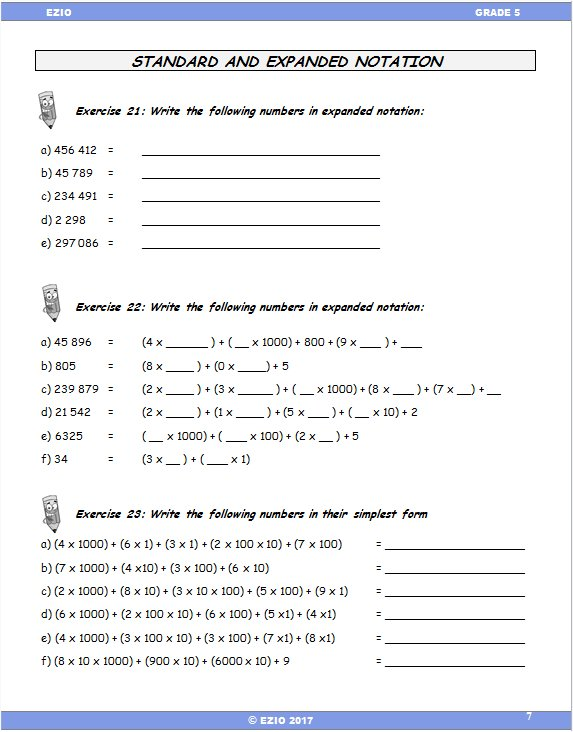Example
Standard and Expanded Notation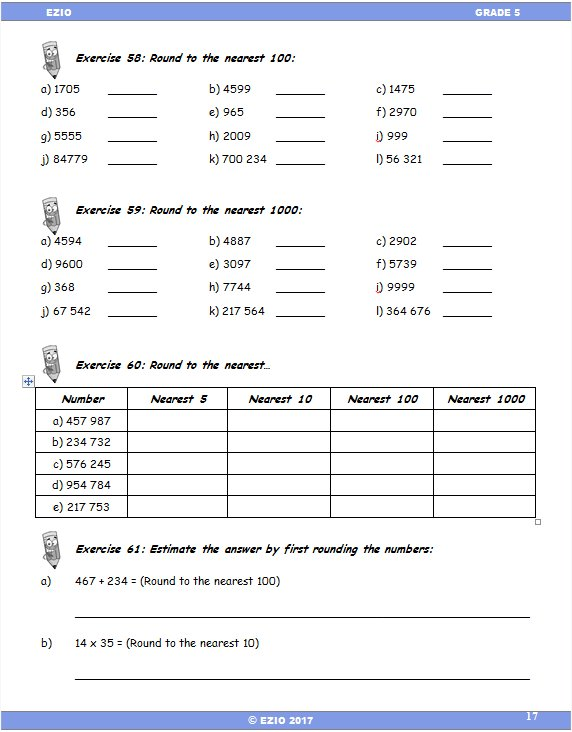Example
Round to nearest 1000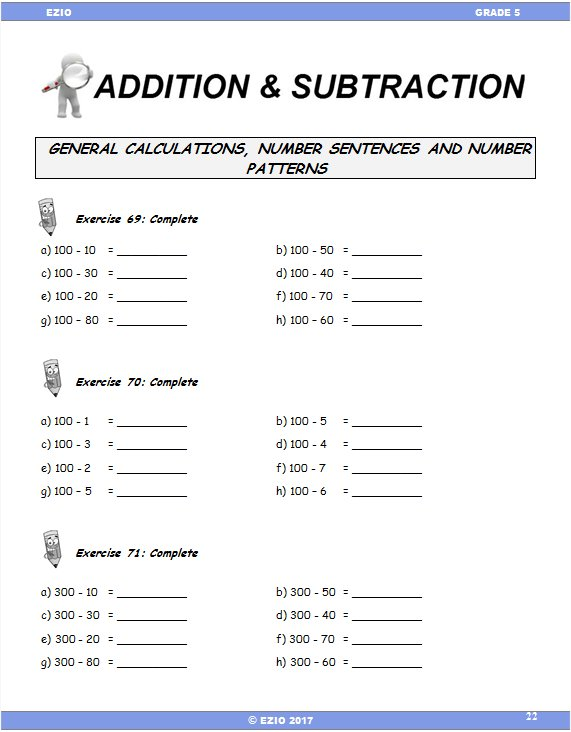Example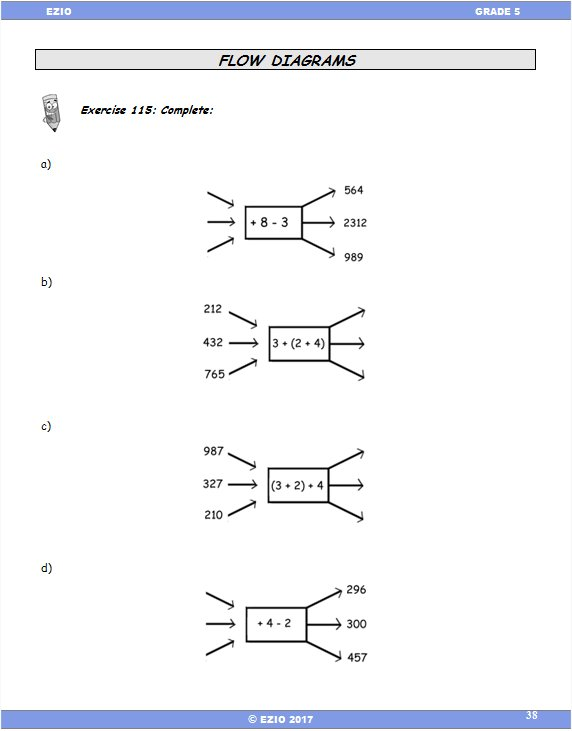Example
Flow Diagrams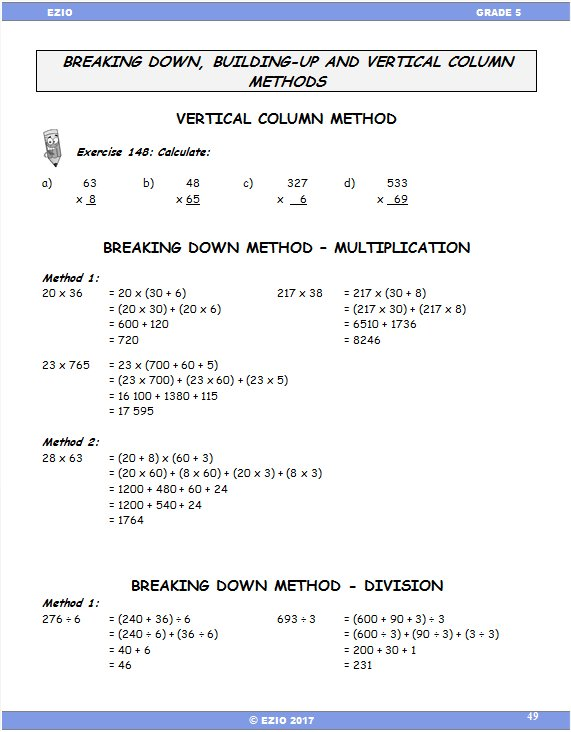Example
Methods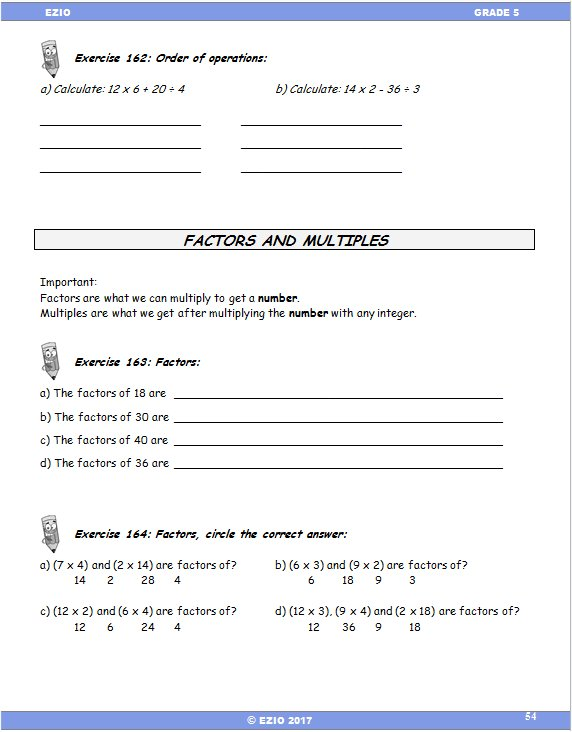Example
Factors and Multiples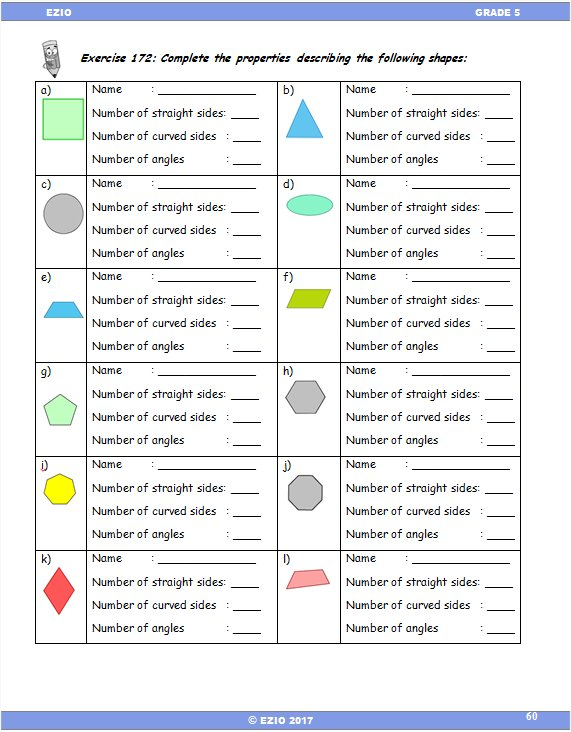Example
Shapes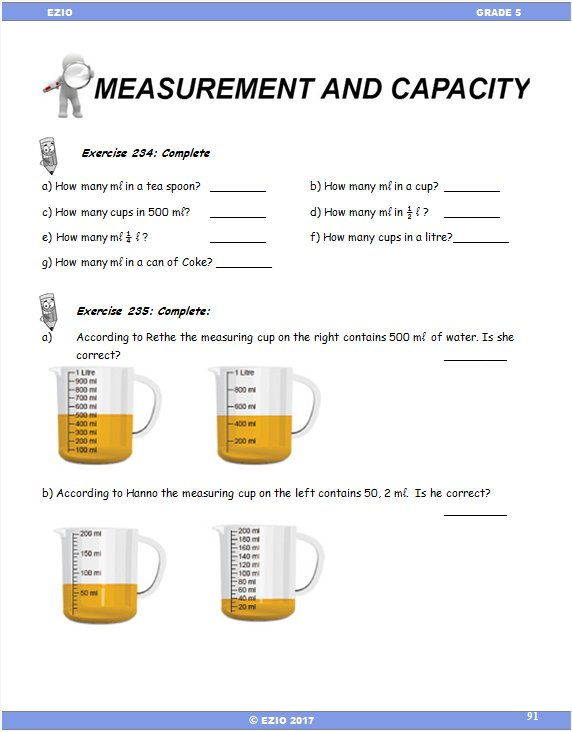Example
Measurement and Capacity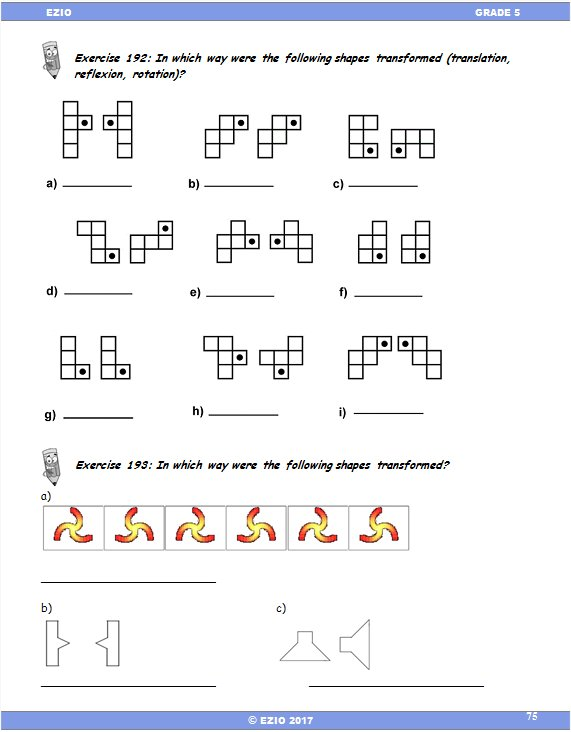Example
Transformation